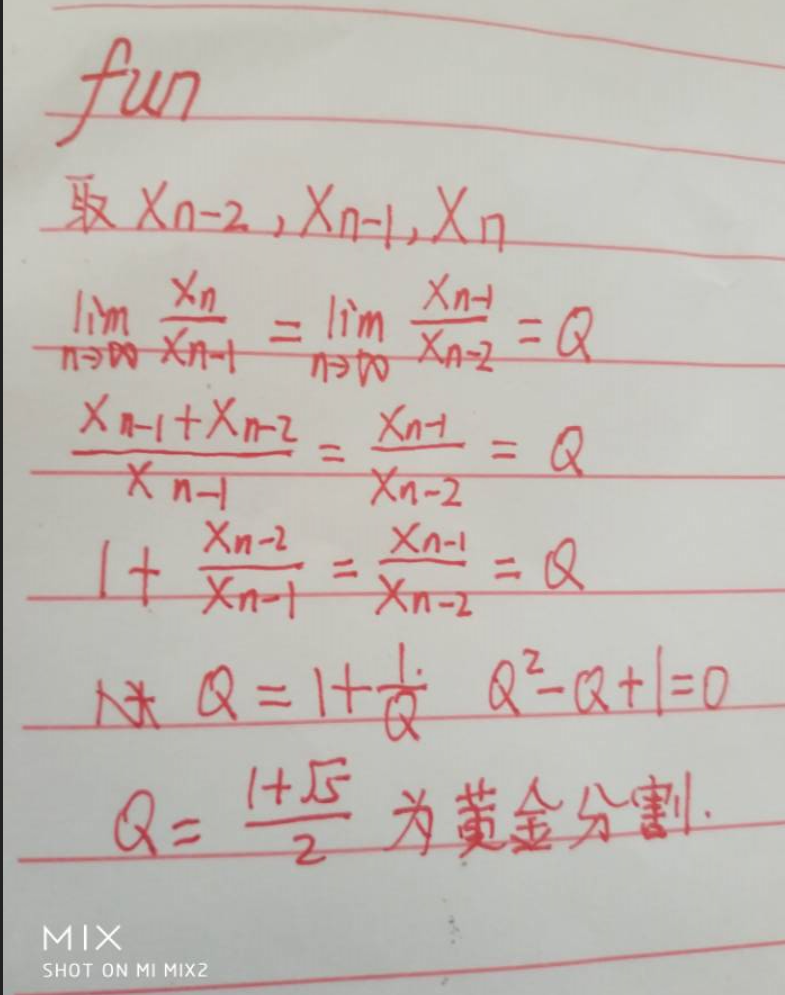# 题目一：

5 2
1 2 3 4 5
6 2
6 5 4 3 2 1

9
9

f(n)=an+min(f(n-1),f(n-2),......f(n-k))

# 题目二：

JiangYu的小金库是一个三维立体的空间，大小为XYZ，里面每个位置都藏着各种价值的宝物，既然是宝物价值自然非同一般，可正可负。

（1 \leq n \leq X \times Y \times Z1≤n≤X×Y×Z)

（1 \leq xi \leq X1≤xi≤X)

（1 \leq yi \leq Y1≤yi≤Y)

（1 \leq zi \leq Z1≤zi≤Z)

（-1e9 \leq ci \leq 1e9−1e9≤ci≤1e9)

2 2 2
8
1 1 1 10
1 1 2 9
1 2 1 10
1 2 2 9
2 1 1 10
2 1 2 9
2 2 1 -1
2 2 2 10

66

https://blog.csdn.net/hebtu666/article/details/82788866这个网址写了详细讲解哦。。。最好要看看

# 题目三：

f(n-1)-(1/2)f(n-3)

# 斐波那契的美# 题目四：

https://blog.csdn.net/hebtu666/article/details/79964233

# 题目五

http://newoj.acmclub.cn/contests/1359/problem/6

#include <bits/stdc++.h>
using namespace std;
int k,n,m,dp;
int a,b;
int pa={0},pb={0};
queue<int> q;
int main()
{
int ak; cin>>ak;
while(ak--)
{
for(int i=1;i<=n;i++)
while(!q[i].empty()) q[i].pop();
scanf("%d%d%d",&k,&n,&m);
memset(dp,0,sizeof(dp));
memset(pa,0,sizeof(pa));
memset(pb,0,sizeof(pb));
for(int i=1;i<=n;i++) cin>>a[i];
for(int i=1;i<=m;i++) cin>>b[i];
for(int i=1;i<=n;i++)
{
q[a[i]].push(i);
if(q[a[i]].size()==k)
{
pa[i]=q[a[i]].front();
q[a[i]].pop();
}
}
for(int i=1;i<=n;i++)
while(!q[i].empty()) q[i].pop();

for(int i=1;i<=m;i++)
{
q[b[i]].push(i);
if(q[b[i]].size()==k)
{
pb[i]=q[b[i]].front();
q[b[i]].pop();
}
}

for(int i=1;i<=n;i++)
{
for(int j=1;j<=m;j++)
{
dp[i][j]=max(dp[i-1][j],dp[i][j-1]);
if(a[i]==b[j] && pa[i]!=0 && pb[j]!=0)
dp[i][j]=dp[pa[i]-1][pb[j]-1]+k;
}
}
printf("%d\n",dp[n][m]);
}
return 0;
}

### 最后总结经验教训：

05-28187

#### 我想参加程序设计竞赛(全国大学生)该做什么准备那?

02-2709-0312万+

#### 三维空间坐标系变换-旋转矩阵

05-25229

#### 2019河北省CCPC 舔狗【贪心】

05-28227

#### 2019CCPC河北省大学生程序设计竞赛 - C - 分治（区间dp）

05-28188

#### 2019CCPC河北省大学生程序设计竞赛 - B - Icebound and Sequence（矩阵分块）

05-26217

#### 2019ccpc河北省赛 A Battle of Balls

05-28362

#### 2019CCPC河北省大学生程序设计竞赛 - L - smart robot（dfs）

05-131377

#### 2019ccpc河北省大学生程序设计竞赛总结

05-26231

#### 2019 河北省赛CCPC C分治©️2020 CSDN 皮肤主题: 书香水墨 设计师: CSDN官方博客点击重新获取扫码支付1.余额是钱包充值的虚拟货币，按照1:1的比例进行支付金额的抵扣。
2.余额无法直接购买下载，可以购买VIP、C币套餐、付费专栏及课程。余额充值